﻿ Digital Circuits and Systems - Circuits i Sistemes Digitals (CSD) - EETAC - UPCBachelor's Degree in Telecommunications Systems and in Network Engineering## Circuits based on logic gates

1. Analysis especifications

Deduce Circuit_Q truth table using method III (Boole's algebra). Check results analysing the same Circuit_Q using method I based on Proteus simulations.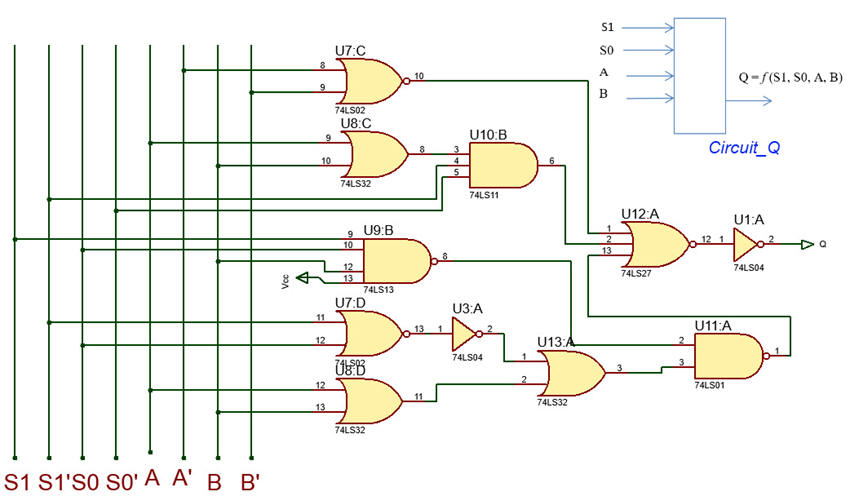Fig. 1. Circuit_Q based on logic gates.

2. Planning the analysis

Analysing Circuit_Q means deducing how it works for every input binary combination represented by its truth table. Fig. 2 shows methods III and method I on how to do it.Fig. 2. Concep map for solving Circuit_Q.

1. To proceed with method III deduce the circuit's equation and apply Boole's algebra to get firstly the SoP or the PoS and secondly, the sum of minterns or product of maxterms, which are conceptually the truth table itself.

2. To test the truth table using method I capture the circuit in Proteus and run simulations.

3. Developing the analysis project

Analysis. Each analysis will be developed using the necessary tools. For instance:

Method III: apply Boolean algebra to deduce the truth table and its minterms or maxterms.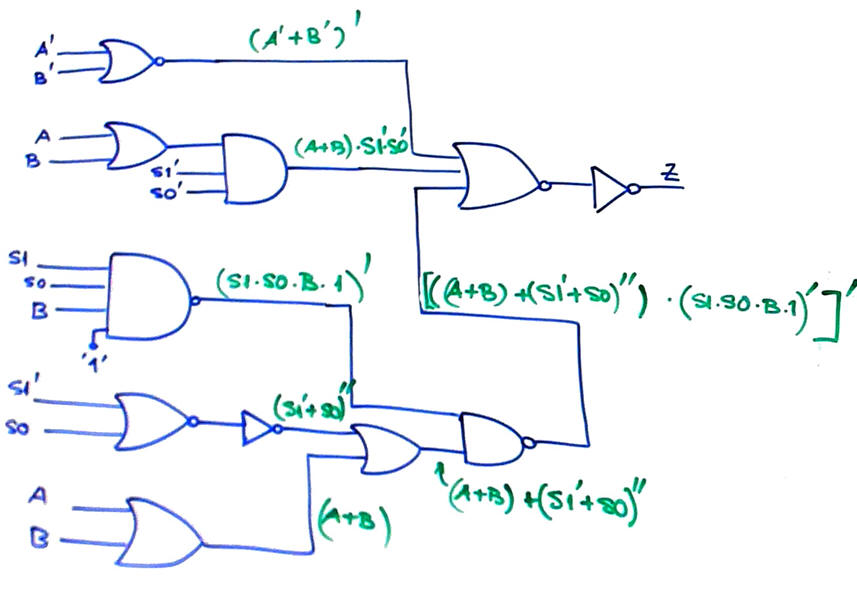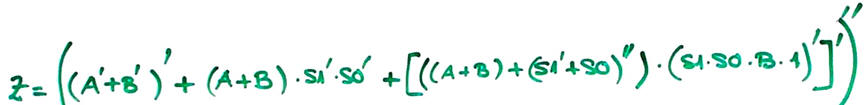Fig. 3.Circuit's algebraic equation.

From the initial circuit equation in Fig. 3 and the simplification process using Boole's algebra, we deduce the circuit's truth table in Fig. 4.Fig. 4. Circuit_Q truth table.

4. Testing and validating analysis results

Method I: Draw Circuit_Q.pdsprj in Proteus using 74LS technology and run all input stimulus.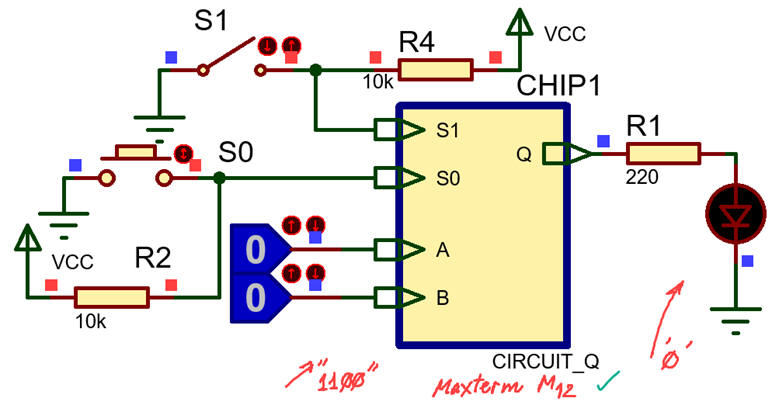Fig. 5. Simulating Circuit_Q in Proteus.

This is another way to verify Circuit_Q using method II WolframAlpha equation.

5. Reporting the analysis

Using pictures, diagrames, sketches, handwritting discussions, etc.

1. Section B: design specifications

We consider the design especifications in Fig. 6 once the analysisi above is completed.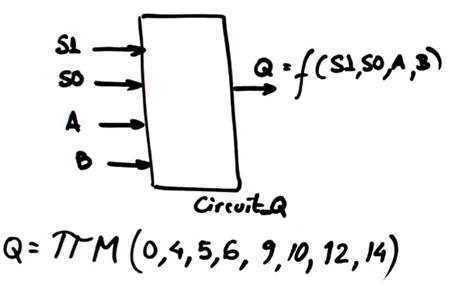Fig. 6. Circuit_Q symbol and truth table deduced from the analysis project above.

- Circuit_Q1. Draw the equivalent circuit using sum of minterms. Check it using  method IV on VHDL.

- Circuit_Q2. Design an equivalent minimised circuit based on PoS. Check it using method II on WolframAlpha

2. Planning the design of equivalent circuits using gates

From the Circuit_Q truth table we can obtain different circuits. For instance:Fig. 7. Concept map for designing alternative circuits from the initial truth table. Set as well project locations.

1. Circuit_Q1. Use the sum of minterns expression to draw the circuit composed of a regular network of BUFFER-NOT - AND - OR. All AND gates (minterms) are 4 inputs. A single OR gate is required. We obtain a regular circuit of three levels of gates.

2. Circuit_Q2. Use to minimise Q = f(S1, S0, A, B) as a SoP and draw the circuit.

3. Design development

Circuit_Q1 is drawn from its initial sum of minterms equation: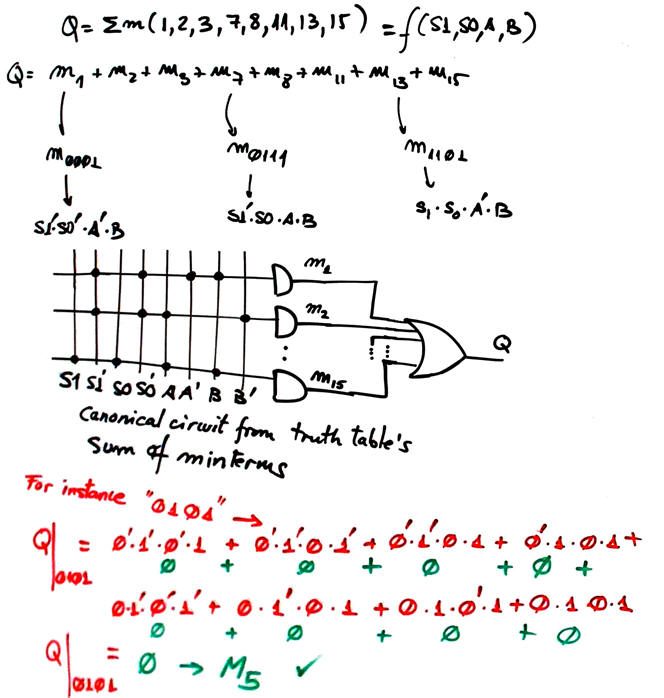Fig. 8. Circuit_Q1 created directly from the sum of minterms canonical equation that defines the truth table.

Circuit_Q2. Run minilog from Circuit_Q2.tbl and draw the circuit based on PoS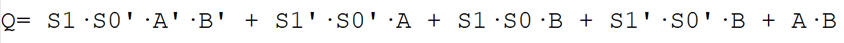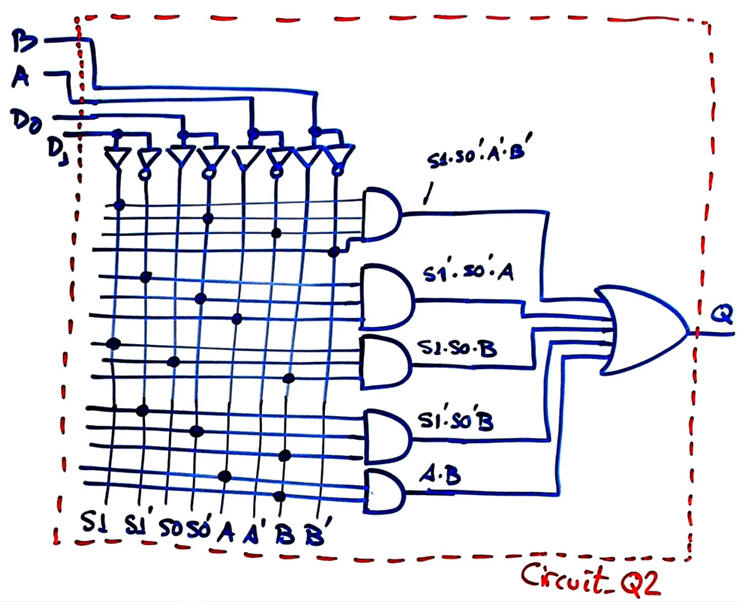Fig. 9. Circuit_Q2 created using the minimised equation SoP.

4. Design testing

Circuit_Q1. Method IV. Let us select a MAX10 10M50DAF484C7 Intel FPGA chip for translating the project to VHDL. Circuit_Q1.vhd.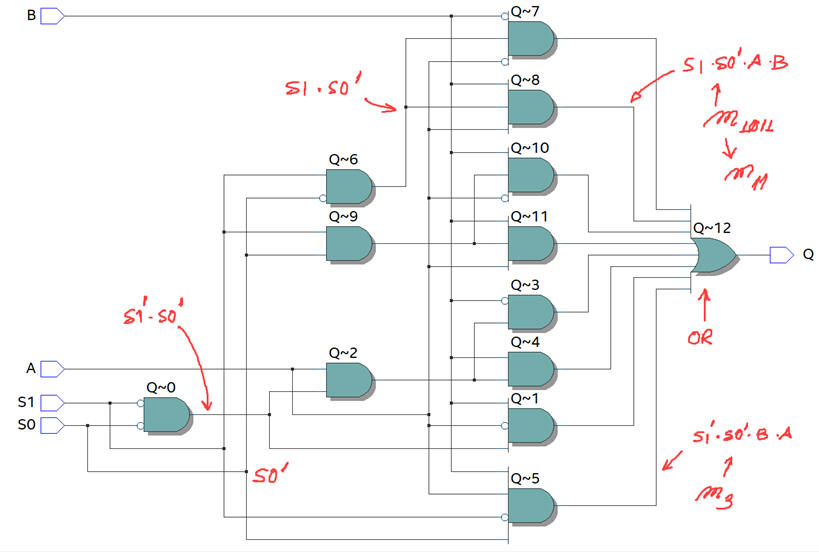Fig. 10. RTL and technology circuits. Be aware how the Quartus Prime embedded synthesiser tool using default parameters implements another simpler equation intead of the sum of minterms ideally described in the RTL view.

Circuit_Q1 is tested using ModelSim functional simulations from this Circuit_Q1_tb.vhd.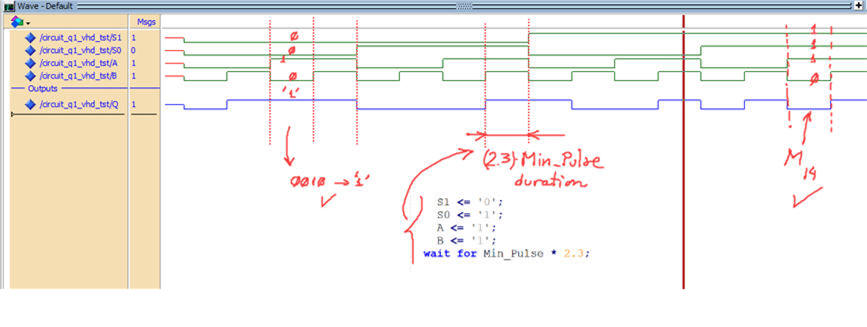Fig. 11. Simulation results for determining and verifying the circuit's truth table. All the input combinations generates

Circuit_Q2. Method II. Let translate the minimised SoP equation to WolframAlpha Circuit_Q2_equ.txt and get results running the engine. Be aware that depending of the variable order when writing the equation, you have to reorder aswell the output table to identify correctly the set of minterms and maxterms.Fig. 12. Table results from WolframAlpha.

5. Reporting the project design

Using pictures, diagrames, sketches, handwritting discussions, etc.